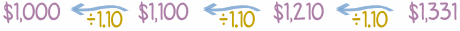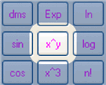# Net Present Value (NPV)Money now is more valuable than money later on.

Why? Because you can use money to make more money!

You could run a business, or buy something now and sell it later for more, or simply put the money in the bank to earn interest.

### Example: Let us say you can get 10% interest on your money.

So \$1,000 now can earn \$1,000 x 10% = \$100 in a year.

Your \$1,000 now becomes \$1,100 next year.So \$1,000 now is the same as \$1,100 next year (at 10% interest):

We say that \$1,100 next year has a Present Value of \$1,000.Because \$1,000 can become \$1,100 in one year (at 10% interest).

If you understand Present Value, you can skip straight to Net Present Value.

Now let us extend this idea further into the future ...

## How to Calculate Future Payments

Let us stay with 10% Interest , which means money grows by 10% every year, like this:So:

• \$1,100 next year is the same as \$1,000 now.
• And \$1,210 in 2 years is the same as \$1,000 now.
• etc

In fact all those amounts are the same (considering when they occur and the 10% interest).

## Easier Calculation

But instead of "adding 10%" to each year it is easier to multiply by 1.10 (explained at Compound Interest):So we get this (same result as above):## Future Back to Now

And to see what money in the future is worth now, go backwards (dividing by 1.10 each year instead of multiplying):### Example: Sam promises you \$500 next year, what is the Present Value?

To take a future payment backwards one year divide by 1.10

So \$500 next year is \$500 ÷ 1.10 = \$454.55 now (to nearest cent).

The Present Value is \$454.55

### Example: Alex promises you \$900 in 3 years, what is the Present Value?

To take a future payment backwards three years divide by 1.10 three times

So \$900 in 3 years is:

\$900 ÷ 1.10 ÷ 1.10 ÷ 1.10
\$900 ÷ (1.10 × 1.10 × 1.10)
\$900 ÷ 1.331
\$676.18 now (to nearest cent).

## Better With Exponents

But instead of \$900 ÷ (1.10 × 1.10 × 1.10) it is better to use exponents (the exponent says how many times to use the number in a multiplication).

### Example: (continued)

The Present Value of \$900 in 3 years (in one go):

\$900 ÷ 1.103 = \$676.18 now (to nearest cent).

And we have in fact just used the formula for Present Value:

PV = FV / (1+r)n

• PV is Present Value
• FV is Future Value
• r is the interest rate (as a decimal, so 0.10, not 10%)
• n is the number of years

### Example: (continued)

Use the formula to calculate Present Value of \$900 in 3 years:

PV = FV / (1+r)n
PV = \$900 / (1 + 0.10)3
PV = \$900 / 1.103
PV = \$676.18 (to nearest cent).Exponents are easier to use, particularly with a calculator. For example 1.106 is quicker than 1.10 × 1.10 × 1.10 × 1.10 × 1.10 × 1.10

## Net Present Value (NPV)

A Net Present Value is when you add and subtract all Present Values:

• Subtract each Present Value you pay

### Example: A friend needs \$500 now, and will pay you back \$570 in a year. Is that a good investment when you can get 10% elsewhere?

Money Out: \$500 now

You invested \$500 now, so PV = -\$500.00

Money In: \$570 next year

PV = \$570 / (1+0.10)1 = \$570 / 1.10 = \$518.18 (to nearest cent)

The Net Amount is:

Net Present Value = \$518.18 - \$500.00 = \$18.18

So, at 10% interest, that investment is worth \$18.18

(In other words it is \$18.18 better than a 10% investment, in today's money.)

A Net Present Value (NPV) that is positive is good (and negative is bad).

But your choice of interest rate can change things!

### Example: Same investment, but try it at 15%.

Money Out: \$500

You invested \$500 now, so PV = -\$500.00

Money In: \$570 next year:

PV = \$570 / (1+0.15)1 = \$570 / 1.15 = = \$495.65 (to nearest cent)

Work out the Net Amount:

Net Present Value = \$495.65 - \$500.00 = -\$4.35

So, at 15% interest, that investment is worth -\$4.35

It is a bad investment. But only because you are demanding it earn 15% (maybe you can get 15% somewhere else at similar risk).

Side Note: the interest rate that makes the NPV zero (in the previous example it is about 14%) is called the Internal Rate of Return.

Let us try a bigger example.

### Example: Invest \$2,000 now, receive 3 yearly payments of \$100 each, plus \$2,500 in the 3rd year. Use 10% Interest Rate.

Let us work year by year (remembering to subtract what you pay out):

• Now: PV = −\$2,000
• Year 1: PV = \$100 / 1.10 = \$90.91
• Year 2: PV = \$100 / 1.102 = \$82.64
• Year 3: PV = \$100 / 1.103 = \$75.13
• Year 3 (final payment): PV = \$2,500 / 1.103 = \$1,878.29

Adding those up gets: NPV = −\$2,000 + \$90.91 + \$82.64 + \$75.13 + \$1,878.29 = \$126.97

Looks like a good investment.

And again, but an interest rate of 6%

### Example: (continued) at a 6% Interest Rate.

• Now: PV = −\$2,000
• Year 1: PV = \$100 / 1.06 = \$94.34
• Year 2: PV = \$100 / 1.062 = \$89.00
• Year 3: PV = \$100 / 1.063 = \$83.96
• Year 3 (final payment): PV = \$2,500 / 1.063 = \$2,099.05

Adding those up gets: NPV = −\$2,000 + \$94.34 + \$89.00 + \$83.96 + \$2,099.05 = \$366.35

Looks even better at 6%

Why is the NPV bigger when the interest rate is lower?Because the interest rate is like the team you are playing against, play an easy team (like a 6% interest rate) and you look good, a tougher team (like a 10% interest) and you don't look so good!

You can actually use the interest rate as a "test" or "hurdle" for your investments: demand that an investment have a positive NPV with, say, 6% interest.

So there you have it: work out the PV (Present Value) of each item, then total them up to get the NPV (Net Present Value), being careful to subtract amounts that go out and add amounts that come in.

And a final note: when comparing investments by NPV, make sure to use the same interest rate for each.